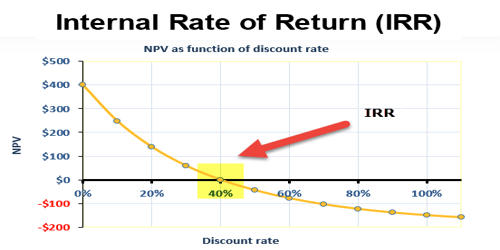Accounting

# Calculation of Internal Rate of Return (IRR)The internal rate of return (IRR) is a metric used in capital budgeting to estimate the profitability of potential investments. Calculating IRR cannot be calculated by hand unless you use a trial and error method until you reach a solution in which the net present value equals zero. Once the internal rate of return is determined, it is typically compared to a company’s hurdle rate or cost of capital.

Calculation of Internal Rate of Return (IRR)

For even case

First, find out the factor:

Factor = Net Cash Outlay(NCO)/Cash flow after tax(CFAT)

The factor is also known as the payback period.

After finding out the factor, locate the factor in the line of annuity table at a given year from row side, if the factor lies exactly in any percentage then that percentage is known as IRR. If the factor does not lie exactly then take two percentages corresponding one higher and another lower and interpolate it.

By interpolation,

IRR= LR + (Factor at LR- Required rate)/(Factor at LR- Factor at HR) x (HR-LR)

where LR = lower rate and HR = higher rate.

For uneven case

First, find out the factor

Factor = NCO/Average CFAT

where, average CFAT= Total sum of CFAT/Total life of the project

After finding the factor, locate the factor in the line of annuity table at a given year from row side, if the factor lies exactly in any percentage then that percentage is known as IRR. If the factor does not lie exactly then take two percentages corresponding one higher and another lower and interpolate it.

By interpolation,

IRR= LR +(TPV at LR – NCO)/(TPV at LR- TPV at HR)x (HR-LR)

Note: For even case, one TPV must be higher than NCO whereas another should be lower than NCO.

Decision Rules Of IRR

If projects are independent

• Accept the project which has a higher IRR than the cost of capital(IRR> k).
• Reject the project which has lower IRR than the cost of capital(IRR

If projects are mutually exclusive

• Accept the project which has higher IRR
• Reject other projects

For the acceptance of the project, IRR must be greater than the cost of capital. Higher IRR is accepted among different alternatives.

Businesses use it to determine which discount rate makes the present value of future after-tax cash flows equal to the initial cost of the capital investment. It is calculated that the net present value of an investment yields zero, and therefore allows the comparison of the performance of unique investments over varying periods of time. It is widely used in analyzing investments for private equity and venture capital, which involves multiple cash investments over the life of a business and cash flow at the end through an IPO or sale of the business.